Note: This document is for an older version of GRASS GIS that will be discontinued soon. You should upgrade, and read the current manual page.

## NAME

v.select - Selects features from vector map (A) by features from other vector map (B).

## KEYWORDS

vector, geometry, spatial query

## SYNOPSIS

v.select
v.select --help
v.select [-tcr] ainput=name [alayer=string] [atype=string[,string,...]] binput=name [blayer=string] [btype=string[,string,...]] output=name operator=string [relate=string] [--overwrite] [--help] [--verbose] [--quiet] [--ui]

### Flags:

-t
Do not create attribute table
-c
Do not skip features without category
-r
Reverse selection
--overwrite
Allow output files to overwrite existing files
--help
Print usage summary
--verbose
Verbose module output
--quiet
Quiet module output
--ui
Force launching GUI dialog

### Parameters:

ainput=name [required]
Name of input vector map
Input vector map from which to select features (A)
alayer=string
Layer number (vector map A)
Vector features can have category values in different layers. This number determines which layer to use. When used with direct OGR access this is the layer name.
Default: 1
atype=string[,string,...]
Feature type (vector map A)
Input feature type
Options: point, line, boundary, centroid, area
Default: point,line,area
binput=name [required]
Name of input vector map
Query vector map (B)
blayer=string
Layer number (vector map B)
Vector features can have category values in different layers. This number determines which layer to use. When used with direct OGR access this is the layer name.
Default: 1
btype=string[,string,...]
Feature type (vector map B)
Input feature type
Options: point, line, boundary, centroid, area
Default: point,line,area
output=name [required]
Name for output vector map
operator=string [required]
Operator defines required relation between features
A feature is written to output if the result of operation 'ainput operator binput' is true. An input feature is considered to be true, if category of given layer is defined.
Options: overlap, equals, disjoint, intersects, touches, crosses, within, contains, overlaps, relate
Default: overlap
overlap: features partially or completely overlap
equals: features are spatially equals (using GEOS)
disjoint: features do not spatially intersect (using GEOS)
intersects: features spatially intersect (using GEOS)
touches: features spatially touches (using GEOS)
crosses: features spatially crosses (using GEOS)
within: feature A is completely inside feature B (using GEOS)
contains: feature B is completely inside feature A (using GEOS)
overlaps: features spatially overlap (using GEOS)
relate: feature A is spatially related to feature B (using GEOS, requires 'relate' option)
relate=string
Intersection Matrix Pattern used for 'relate' operator

## DESCRIPTION

v.select allows the user to select features from a vector map by features from another one.

Supported operators (without GEOS; using GRASS' own algorithm):

• overlap - features partially or completely overlap (GEOS equivalent: intersects)
Supported operators (internally using GEOS - Geometry Engine, Open Source):
• equals - features are spatially equals
• disjoint - features do not spatially intersect
• intersects - features spatially intersect (equivalent to native 'overlap')
• touches - features spatially touches
• crosses - features spatially crosses
• within - feature A is completely inside feature B
• contains - feature B is completely inside feature A
• overlaps - features spatially overlap
• relate - feature A is spatially related to feature B

## NOTES

Only features with category numbers will be considered. If required the v.category module can be used to add them. Typically boundaries do not need to be given a category number, as an area's attributes are inherited from the centroid. Typically points, lines, and centroids will always want to have a cat number. E.g. take a road which separates two farms. It is ambiguous as to which farm an attribute that is attached to the road belongs to. The boundary only needs a cat number if it will hold its own attributes, such as road name or pavement form. A centroid in each paddock holds the information with respect to ownership, area, etc.

## EXAMPLES

Preparation of example data (North Carolina sample dataset):
```# Create an grid for overlaying to ZIP code vector map
v.mkgrid map=boxgrid grid=10,10 position=coor \
coordinates=583600,201500 box=5000,5000

# set region to ZIP codes and boxgrid vector maps
g.region vector=zipcodes_wake,boxgrid -p res=100 -a
# enlarge region a bit for "white border" around map in monitor
g.region n=n+1000 s=s-1000 w=w-1000 e=e+1000 -p
d.mon wx0
```

### OVERLAP: features partially or completely overlap (using GRASS)

Select grid boxes (North Carolina sample dataset):
```d.vect map=zipcodes_wake fill_color=0:128:0
d.vect map=boxgrid fill_color=85:130:176
v.select ainput=boxgrid binput=zipcodes_wake output=v_select_OVERLAP operator=overlap
d.vect map=v_select_OVERLAP
d.vect map=zipcodes_wake type=boundary color=255:255:50
```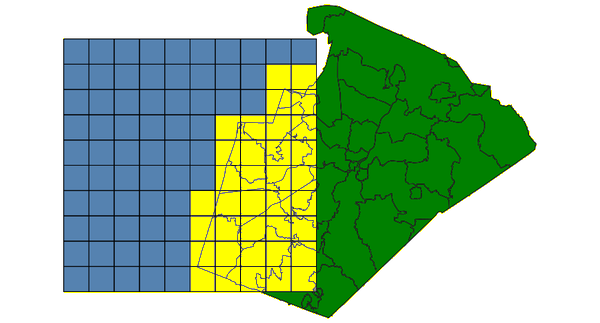v.select with OVERLAP operator: selected grid boxes shown in yellow (using GRASS method)

### OVERLAPS features spatially overlap (using GEOS)

Select grid boxes (North Carolina sample dataset):
```d.vect map=zipcodes_wake fill_color=0:128:0
d.vect map=boxgrid fill_color=85:130:176
v.select ainput=boxgrid binput=zipcodes_wake output=v_select_OVERLAPS operator=overlaps
d.vect map=v_select_OVERLAPS
d.vect map=zipcodes_wake type=boundary color=255:255:50
```v.select with OVERLAPS operator: selected grid boxes shown in yellow (using GEOS method)

### DISJOINT: features do not spatially intersect (using GEOS)

Select grid boxes (North Carolina sample dataset):
```d.vect map=zipcodes_wake fill_color=0:128:0
d.vect map=boxgrid fill_color=85:130:176
v.select ainput=boxgrid binput=zipcodes_wake output=v_select_DISJOINT operator=disjoint
d.vect map=v_select_DISJOINT
d.vect map=zipcodes_wake type=boundary color=255:255:50
```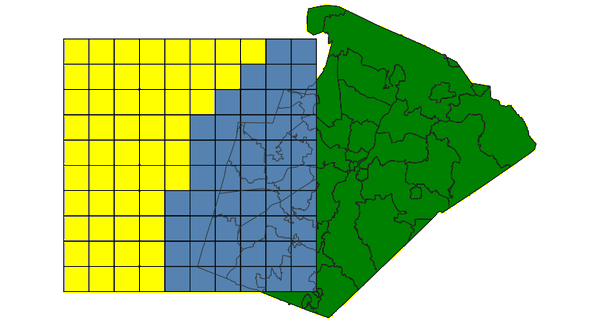v.select with DISJOINT operator: selected grid boxes shown in yellow

### EQUALS: features are spatially equals (using GEOS)

Select zipcode polygon (North Carolina sample dataset):
```d.vect map=zipcodes_wake fill_color=0:128:0
v.extract input=zipcodes_wake where=ZIPCODE_ID=35 output=zipcodeID35
v.select ainput=zipcodes_wake binput=zipcodeID35 output=v_select_EQUALS operator=equals
d.vect map=v_select_EQUALS
d.vect map=zipcodes_wake type=boundary color=255:255:50
```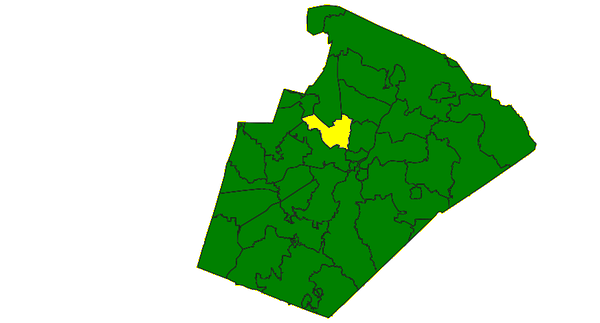v.select with EQUALS operator: selected grid boxes shown in yellow

### INTERSECTS: features spatially intersect (using GEOS)

Select zipcode polygons (North Carolina sample dataset):
```d.vect map=zipcodes_wake fill_color=0:128:0
d.vect map=boxgrid fill_color=85:130:176
v.select ainput=zipcodes_wake binput=boxgrid output=v_select_INTERSECTS operator=intersects
d.vect map=v_select_INTERSECTS
d.vect map=boxgrid type=boundary color=255:255:50
```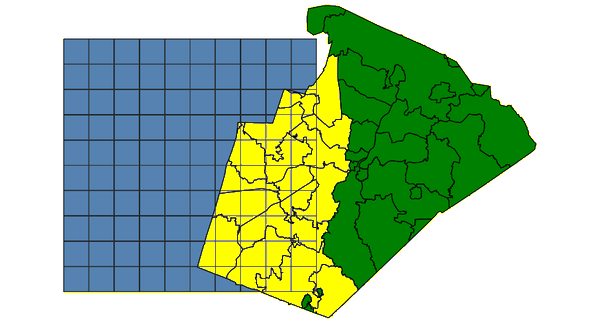v.select with INTERSECTS operator: selected grid boxes shown in yellow

### TOUCHES: features spatially touches (using GEOS)

Select polygons (North Carolina sample dataset):
```d.vect map=zipcodes_wake fill_color=0:128:0
d.vect map=zipcodeID35 fill_color=85:130:176
v.select ainput=zipcodes_wake binput=zipcodeID35 output=v_select_TOUCHES operator=touches
d.vect map=v_select_TOUCHES
d.vect map=zipcodes_wake type=boundary color=255:255:50
```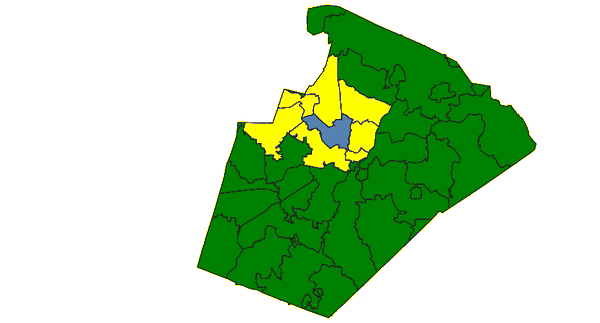v.select with TOUCHES operator: selected polygons shown in yellow (blue: input polygon)

### CROSSES: features spatially crosses (using GEOS)

Select zipcode polygons by lines (North Carolina sample dataset):
```d.vect map=zipcodes_wake fill_color=0:128:0
d.vect map=busroute1 color=200:27:27 width=3
v.select ainput=zipcodes_wake binput=busroute1 output=v_select_CROSSES operator=crosses
d.vect map=v_select_CROSSES
d.vect map=zipcodes_wake type=boundary color=255:255:50
d.vect map=busroute1 color=200:27:27 width=3
```v.select with CROSSES operator: selected polygons shown in yellow (red: input lines)

### WITHIN feature A is completely inside feature B (using GEOS)

Select zipcode polygons (North Carolina sample dataset):
```d.vect map=zipcodes_wake fill_color=0:128:0
d.vect map=boundary_county fill_color=85:130:176
v.select ainput=zipcodes_wake binput=boundary_county output=v_select_WITHIN operator=within
d.vect map=v_select_WITHIN
```v.select with WITHIN operator: selected polygons shown in yellow (blue: input polygons)

### CONTAINS feature B is completely inside feature A (using GEOS)

Select zipcode polygon (North Carolina sample dataset):

#### CONTAINS with polygons

```d.vect map=zipcodes_wake fill_color=0:128:0
d.vect map=zipcodeID35 fill_color=85:130:176
v.select ainput=zipcodes_wake binput=zipcodeID35 \
output=v_select_CONTAINS_pol operator=contains
d.vect map=v_select_CONTAINS
```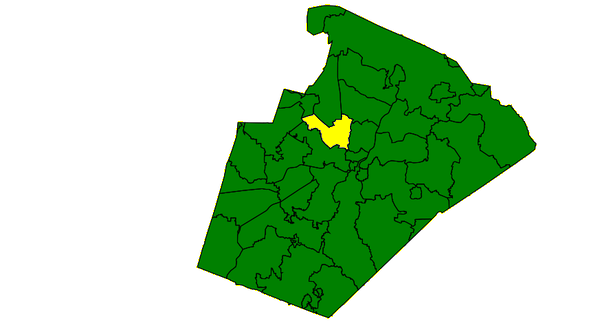v.select with CONTAINS operator: selected polygon shown in yellow (blue: input polygon, not visible)

#### CONTAINS with points

```d.vect map=zipcodes_wake fill_color=0:128:0
d.vect map=hospitals fill_color=195:31:31 icon=basic/cross3 size=10
v.select ainput=zipcodes_wake binput=hospitals \
output=v_select_CONTAINS_pnts operator=contains
d.vect map=v_select_CONTAINS_pnts
d.vect map=hospitals fill_color=195:31:31 icon=basic/cross3 size=10
```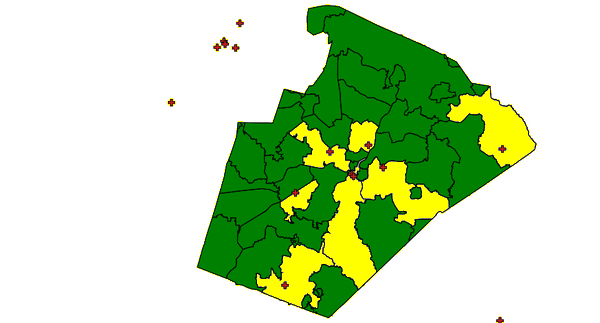v.select with CONTAINS operator: selected polygons shown in yellow (red: input points)

### RELATE feature A is spatially related to feature B (using GEOS)

This operator additionally requires the relate parameter (in other GIS called 'ST_Relate'). This operator allows calculating the Dimensionally Extended nine-Intersection Model (DE-9IM). In the following one example: Select polygon with 'TOUCHES' operator (North Carolina sample dataset):
```d.vect map=zipcodes_wake fill_color=0:128:0
d.vect map=zipcodeID35 fill_color=85:130:176
v.select ainput=zipcodeID35 binput=zipcodes_wake \
output=v_select_TOUCHES_relate operator=relate relate='T********'
d.vect map=v_select_TOUCHES
```
The result of relate='T********' is the same as seen above in the example 'TOUCHES'. See the DE-9IM page for related operators and their definition.

### Extraction of points falling into a polygon

Extract fire stations (points) falling into urban area (polygon) - North Carolina data set (point in polygon test):
```v.select ainput=firestations binput=urbanarea output=urban_firestations \
operator=overlap
```

### Extraction of lines overlapping with a polygon

Extract railroad lines from zip code map overlapping with the urban area (line in polygon test):
```v.select ainput=railroads binput=urbanarea \
```

### Extraction of areas overlapping with a line

Extract those areas from zip code map which overlap with railroads (polygon on line test):
```# first add a tiny buffer around railroad lines:
distance=20

v.select ainput=zipcodes_wake binput=railroads_buf20m \
```

v.category, v.clip, v.overlay, v.extract

GRASS SQL interface
GEOS - Geometry Engine, Open Source